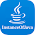» » Encapsulation

Encapsulation

Need for Encapsulation

• Any program of  any programming language contains only two parts.
i.Data
ii.Logic
• Out of these two highest priority would be always given to data part.
• Functions /logics would e giving output based on the data.
• There is no proper security for the data part in the structured programming language programs like c - program.
• There was no proper separation between the data and functions of different domains because of which complexity of the programming increases and data insecurity arises
• The concept of proper binding is missing in structured programming languages

Reason for Data Insecurity in C-Programing language:

• It is the  GLOBAL VARIABLES which leads to the data insecurity in c- programs
• GLOBAL VARIABLES would be available for both related and unrelated functions also.
• Whenever an unrelated function accesses the data in a wrong way data corruption arises

Sample c -program:

1. #include <stdio.h>
2.
3. /* global variable declaration */
4. int a = 20;
5.
6. int main ()
7. {
8.
9. /* local variable declaration in main function */
10.   int a = 10;
11.   int b = 20;
12.   int c = 0;
13. int mul=0;
14.
15.  c = sum( a, b);
16.
17.  printf ("a+b = %d\n",  c);
18.
19. mul= sum( a, b);
20.
21. printf ("a*b= %d\n",  mul);
22. return 0;
23.
24. /* function to add two integers */
25. int sum(int a, int b)
26. {
27.     return a + b;
28. }
29.
30. /* function to multiply two integers */
31. int mul(int a, int b)
32. {
33.     return a * b;
34. }

What is Encapsulation?

• The concept of binding the data along with its related and corresponding functions is known as Encapsulation.

Binding:

• The concept of restricting the availability of the data only to its related areas and related functions it is known as binding.
• Because of binding we can restrict the availability of dta to the unrelated functions
• So that unrelated functions cannot access the data
• Class is the base for encapsulation

Example program:

1. package com.instanceofjava;
2.
3. public class Student {
4.
5. // variables
6.    int rno;
7.     String name;
8.     String clas;
10.     int rank;
11.
12. //setter and getter methods of variables
13.
14.  public int getRno() {
15.        return rno;
16.     }
17.
18. public void setRno(int rno) {
19.        this.rno = rno;
20. }
21.
22. public String getName() {
23.         return name;
24. }
25.
26.  public void setName(String name) {
27.         this.name = name;
28. }
29.
30. public String getClas() {
31.        return clas;
32. }
33.
34. public void setClas(String clas) {
35.         this.clas = clas;
36. }
37.
38. public String getAddress() {
40. }
41.
44. }
45.
46. public int getRank() {
47.     return rank;
48.  }
49.
50. public void setRank(int rank) {
51.         this.rank = rank;
52.  }
53.
54.     //constructor
55.    Student(int rno,String name,String clas,String Address,int rank){
56.
57.         this.rno=rno;
58.         this.name=name;
59.         this.clas=clas;
60.
62.         this.rank=rank;
63.
64.     }
65.
66.  public static void main(String args[]){
67.
68.     Student student= new Student(1, "sai", "class IV", "hyderabad", 1);
69.
70.     System.out.println("Name: "+student.getName());
71.     System.out.println("Class: "+student.getClas());
72.     System.out.println("Rno: "+student.getRno());
73.
74.     System.out.println("Rank: "+student.getRank());
76.
77.   }
78. }

Output:

1. Name: sai
2. Class: class IV
3. Rno: 1
4. Rank: 1

Instance Of Java

We will help you in learning.Please leave your comments and suggestions in comment section. if you any doubts please use search box provided right side. Search there for answers thank you.

4 comments for Encapsulation

1.In the program if explain where the bending is happening then new learners can easily understand.

1.Yeah, even I too have the same question. I am new in Java. Though I understood the concept clearly but I am not getting exactly where binding is happening in the program.

2.Keeping variables and corresponding methods in one place== class==binding happening

2.Good explanation.